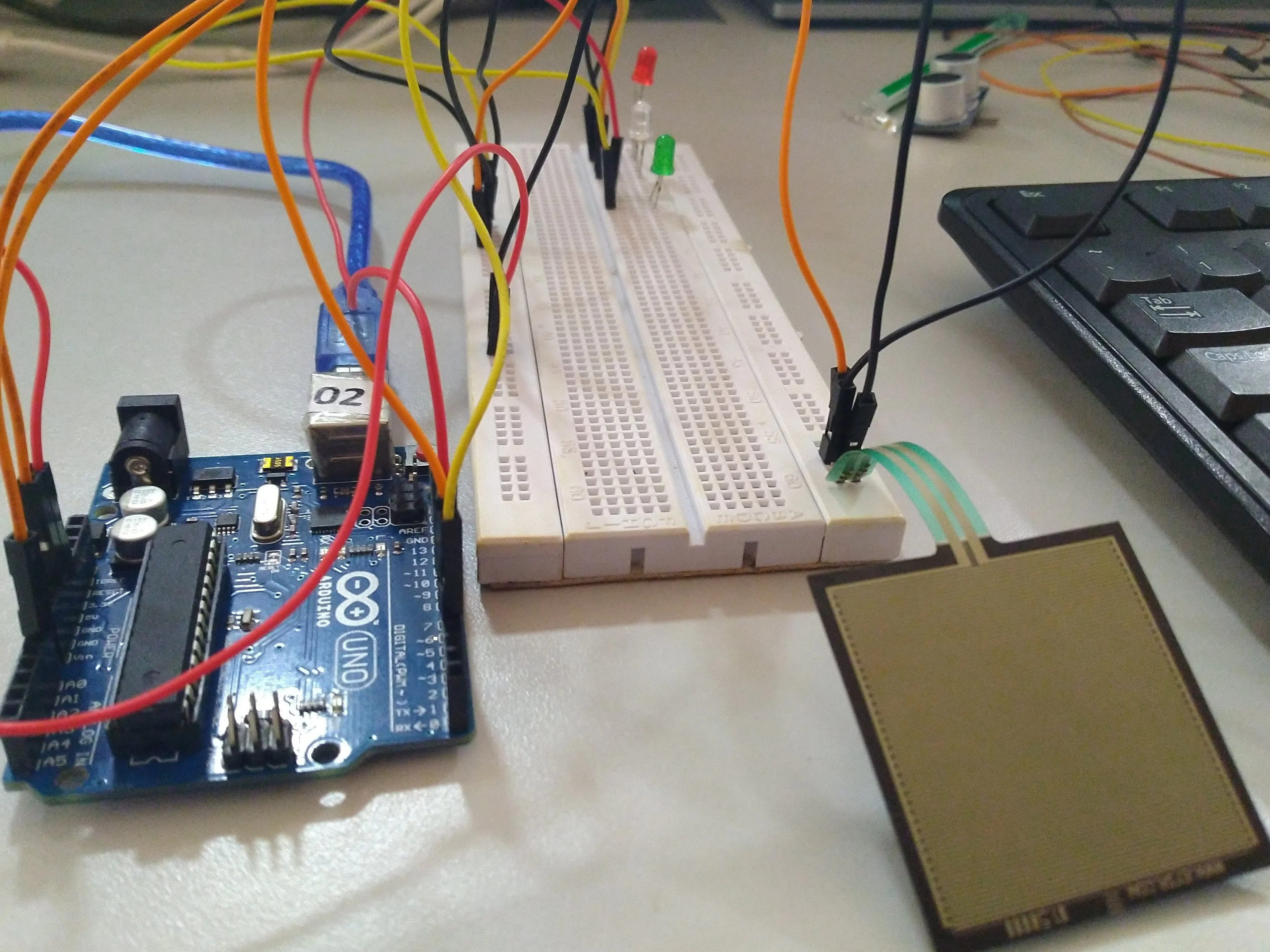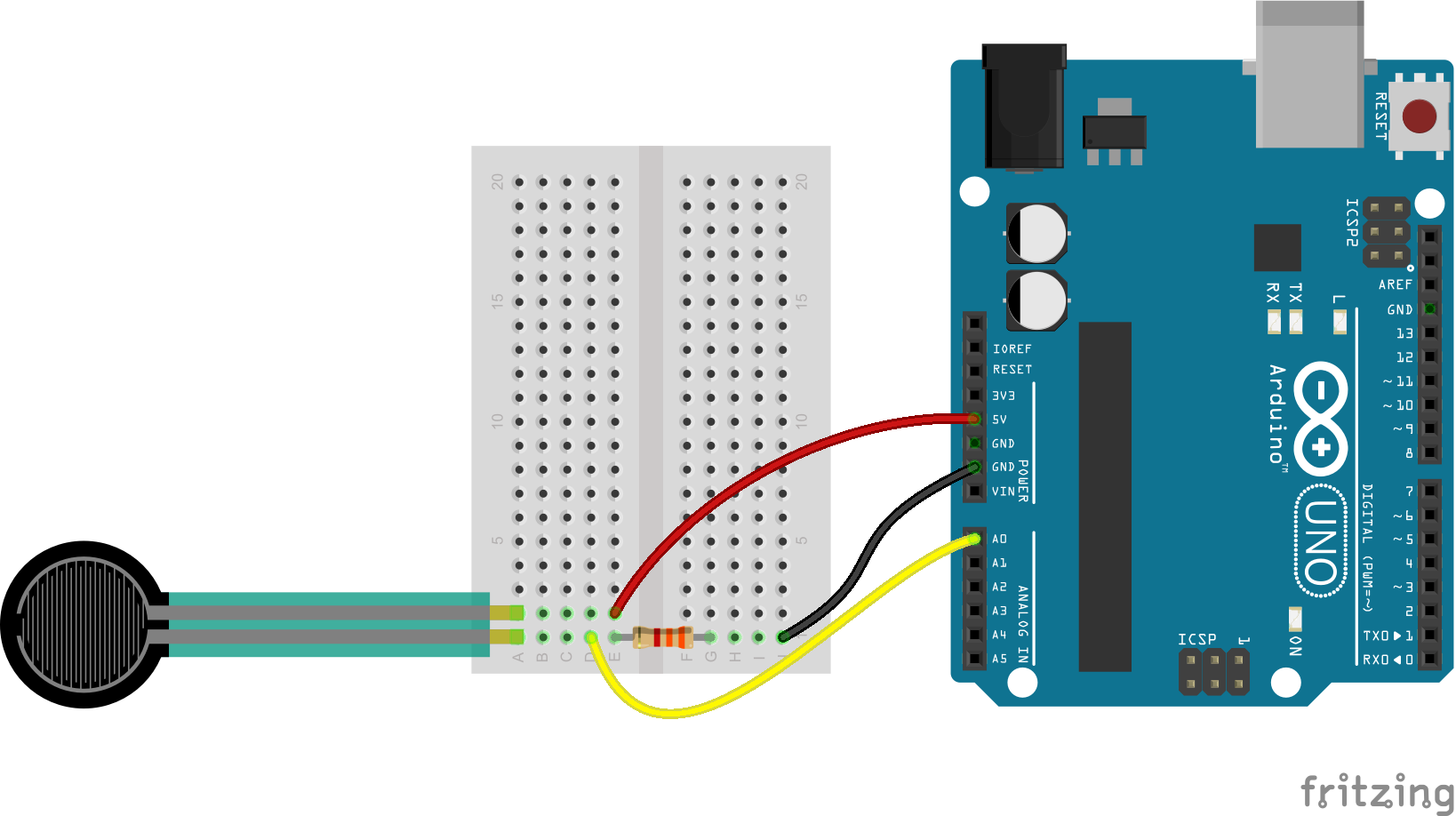Project tutorial# Pressure Pad Interfacing with Arduino

Glowing LED to detect the increase in pressure by pressure pad using Arduino.

• 36,853 views
• 20 respects

## Apps and online services

Pressure pads are designed to detect when pressure is applied to an area, and they come in a variety of quality and accuracy. The simplest pressure pads are often the equivalent of big switches. Inside a pressure pad are two layers of foil separated by a layer of foam with holes in it. When the foam is squashed, the metal contacts touch through the foam and complete the circuit.

This tutorial serves as a quick primer on rressure pads and demonstrates how to hook them up and use them with Arduino.

## Code

```const int FSR_PIN = A0; // Pin connected to FSR/resistor divider
const int LED_PIN1 = 7;
const int LED_PIN2 = 6;
const int LED_PIN3 = 4;
const float VCC = 4.98; // Measured voltage of Ardunio 5V line
const float R_DIV = 3230.0; // Measured resistance of 3.3k resistor

void setup()
{
Serial.begin(9600);
pinMode(FSR_PIN, INPUT);
pinMode(LED_PIN1, OUTPUT);
pinMode(LED_PIN2, OUTPUT);
pinMode(LED_PIN3, OUTPUT);
}

void loop()
{
// If the FSR has no pressure, the resistance will be
// near infinite. So the voltage should be near 0.
{
float fsrV = fsrADC * VCC / 1023.0;
// Use voltage and static resistor value to
// calculate FSR resistance:
float fsrR = R_DIV * (VCC / fsrV - 1.0);
Serial.println("Resistance: " + String(fsrR) + " ohms");
// Guesstimate force based on slopes in figure 3 of
// FSR datasheet:
float force;
float fsrG = 1.0 / fsrR; // Calculate conductance
// Break parabolic curve down into two linear slopes:
if (fsrR <= 600)
force = (fsrG - 0.00075) / 0.00000032639;
else
force =  fsrG / 0.000000642857;
Serial.println("Force: " + String(force) + " g");
Serial.println();
if(force<10)
{
digitalWrite(LED_PIN1,LOW);
digitalWrite(LED_PIN2,LOW);
digitalWrite(LED_PIN3,LOW);}
if(force>20)
{
digitalWrite(LED_PIN1,HIGH);
digitalWrite(LED_PIN2,LOW);
digitalWrite(LED_PIN3,LOW);

}
if(force>60)
{
digitalWrite(LED_PIN2,HIGH);
digitalWrite(LED_PIN1,LOW);
digitalWrite(LED_PIN3,LOW);
}
if(force>100)
{
digitalWrite(LED_PIN3,HIGH);
digitalWrite(LED_PIN2,LOW);
digitalWrite(LED_PIN1,LOW);
}

delay(500);

}
else
{
// No pressure detected
}
}
```

## Schematics#### How to make a thermometer with Arduino and the LM35 sensor

Project tutorial by Moreira

• 1,505 views
• 2 respects

• 14,797 views
• 7 respects

#### The Vibrating Pressure Temperature Altitude Tilt Detector

Project tutorial by Kutluhan Aktar

• 3,767 views
• 6 respects

#### Create a Diving Computer with an MS5803–01BA Pressure Sensor

Project showcase by Varul Jain

• 4,050 views
• 4 respects

#### nRF24L01 Interfacing with Arduino | Wireless Communication

by Aqib

• 172,298 views